# What will you do?

You're in place of Dhoni and you have to score 6 runs in 3 balls.

You cannot change the strike. This means you can only score 2, 4 or 6 runs off a ball.

There are players along the boundary line, hence you cannot hit a 4.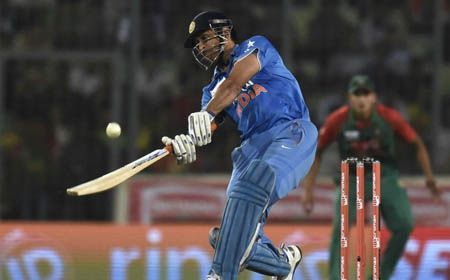What are the different ways in which you can complete the runs required and win the match?

# Scoring the Winning Runs.

We can score 6 runs by:

2 x 3 = 6
Or
6 x 1 = 6
Or 3 x 2 = 6

As we can see, the number 6 is represented as a product of two numbers here, 2 and 3.

When a number is represented as the product of two smaller numbers, the smaller numbers are called factors of the number.

In this case, since, 2 x 3 = 6 we can say that 2 and 3 are factors of 6.

Also, 1 x 6 = 6, hence, we can say 1 and 6 are factors of 6.

# Factors.

When a number is represented as the product of two smaller numbers, the smaller numbers are called factors of the number.

In this case, since, 2 x 3 = 6 we can say that 2 and 3 are factors of 6.

Also, 1 x 6 = 6, hence, we can say 1 and 6 are factors of 6.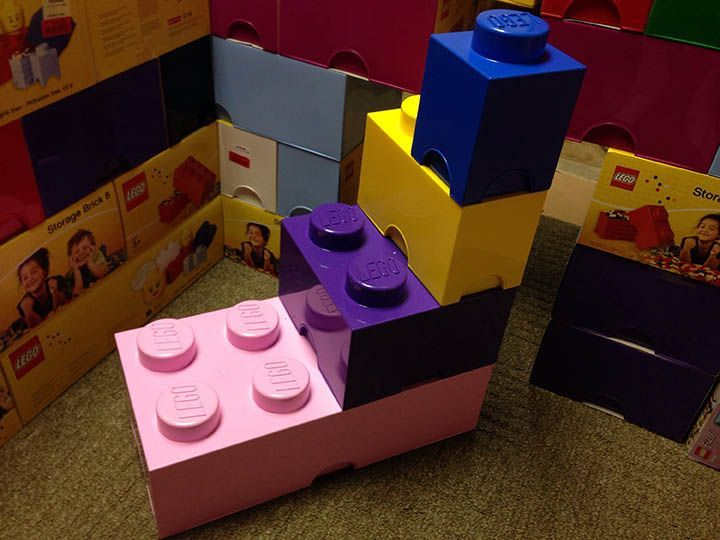The image has a 8 dot lego brick, 4 block lego brick, 2 block lego brick and a 1 block lego brick.

All of the smaller bricks are factors of the larger lego brick.

That is, 1 is a factor of 2, 1 and 2 are factors of 4, 1, 2 and 4 are factors of 8.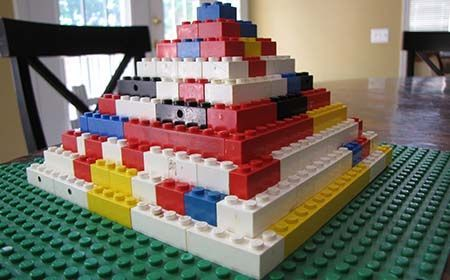When we know about factors we can create such beautiful structures.

# Access All Content

## More from Grade 4 Math# Pictograph

Representing your world in pictures! See how the type and number of a quantity can be mathematically represented pictorially.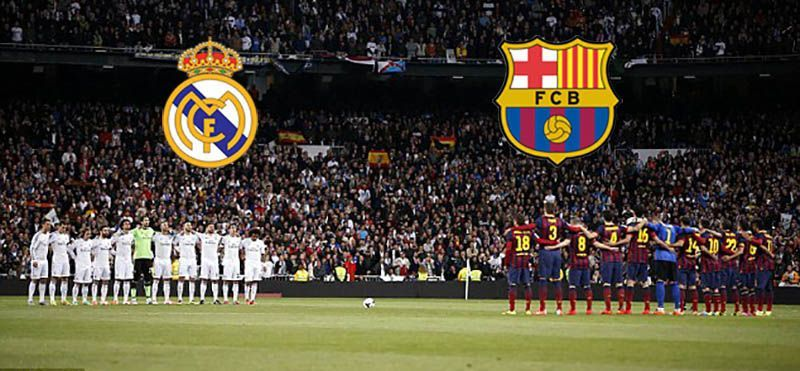# Venn Diagram

The easiest way to imagine sets visually. See the power of Venn Diagrams.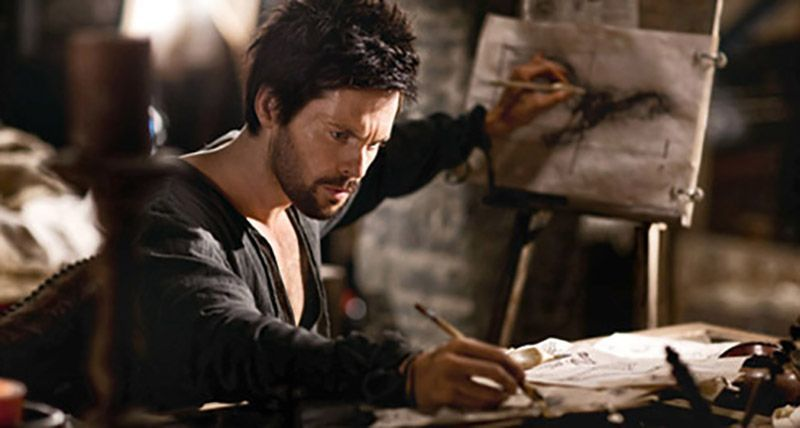# Venn Diagram Example

Learn how to use Venn Diagrams to solve real world problems with these interesting examples.